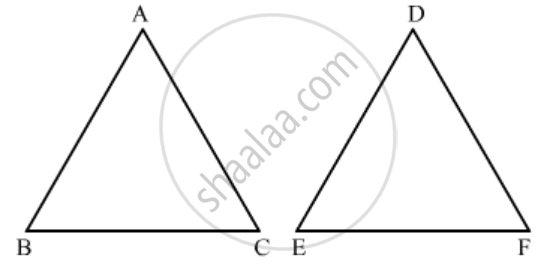Advertisement Remove all ads

# If in Two Triangles Abc and Def, a B D E = B C F E = C a F D , Then (A) ∆Fde ∼ ∆Cab (B) ∆Fde ∼ ∆Abc (C) ∆Cba ∼ ∆Fde (D) ∆Bca ∼ ∆Fde - Mathematics

MCQ

If in two triangles ABC and DEF, $\frac{AB}{DE} = \frac{BC}{FE} = \frac{CA}{FD}$, then

#### Options

• ∆FDE ∼ ∆CAB

• ∆FDE ∼ ∆ABC

• ∆CBA ∼ ∆FDE

• ∆BCA ∼ ∆FDE

Advertisement Remove all ads

#### Solution

We know that if two triangles are similar if their corresponding sides are proportional.It is given that ΔABC and ΔDEF are two triangles such that

(AB)/(DE)=(BC)/(EF)=(CA)/(FD).

$\angle A = \angle D$

$\angle B = \angle E$

$\angle C = \angle F$

∴ ΔCAB ∼ ΔFDE

Hence the correct answer is a.

Concept: Triangles Examples and Solutions
Is there an error in this question or solution?
Advertisement Remove all ads

#### APPEARS IN

RD Sharma Class 10 Maths
Chapter 7 Triangles
Q 20 | Page 133
Advertisement Remove all ads

#### Video TutorialsVIEW ALL 

Advertisement Remove all ads
Share
Notifications

View all notifications

Forgot password?
Course